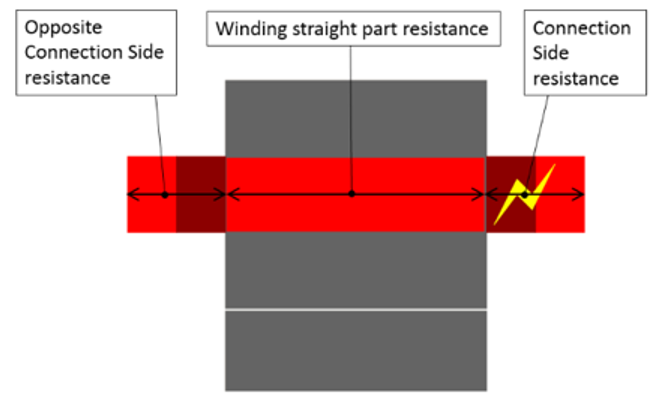# Resistances

## 1. Resistances – Resistance at 20°C and at ref. temperature

 Label Symbol Tooltip, note, formula Phase resistance * Phase resistance Line-Line resistance * Line-Line resistance Parallel path number Number of parallel paths Parallel path resistance Value of parallel path resistance Winding straight part resistance *End-winding resistance * Connection side end-winding resistance * Opposite connection side end-winding resistance *
Note: The reference temperature is a user input parameter defined in the winding – X-Factor tab.
Note: The connection side end-winding resistance considers the additional length corresponding to the connections between coils.
Note: For each parallel path, the resistances are computed and displayed for the winding straight part, the end-winding part ( at connection side and at opposite connection side)